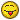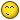# Bpm To Ms Problems

I have a track that is 110 BPM
I converted that over to MSecs by 60000/110 (545.455)
I then Divided that by 8 to give me the MS of each tick and then multiplied that by 64 to give me the pattern length in ms (4363.64)
When I go to File>Render to Disk>Selection in Sequence I can see that my song has 45 patterns
I then multiply the length in MS * 45 and get the legnth of the song in MS (196363.8)

The formula I am using is:
(((60000/BPM)/8)*64)*PatternLength = Length of Track in MS

Something is wrong, because when I convert this over to Mins and Secs it doesn’t line up with the mins and secs of the track I’ve just exported.
I’m just hoping someone might be able to see what I’ve done wrong. I’ve double checked and I’m not swinging the track or changing the BPM’s.

Thanks again you guys,
Hunz

Is it off by a tiny amount, a huge amount, or what? It would be useful to know the length of the exported track and get an idea of exactly how wrong/different things are.

For calc bpm to ms I use native Renoise delay, but it’s has limit to 2 sec…

Bantai and Conner were doing some various algorithm concepts regarding the XRNS midi export, perhaps this info might help you:

Hey,

3:16.363 is the final output on the Re-noise track
3.27.27 is where I end up

I’ve been working backwards for a bit to see if I’ve messed something up (which I know I have). I’m not sure if things have a ‘0’ count so that could help here and there.

Thanks Dblue.
Hunz.

It’s also quite inaccurate since it rounds to the nearest whole millisecond, so you could potentially introduce a lot of error into your final results. The actual calculations themselves are very simple to begin with, so it’s a waste of time using the delay effect for this if you are trying to calculate any meaningful results.

seconds per beat = 60 / beats per minute

milliseconds per beat = 60000 / beats per minute

Something else I thought about: is the song possibly an old format RNS which used the older (and slightly inaccurate) timing methods, or is it a modern XRNS?

Thank you. Am looking over it all nowHunz

It’s all been written in 2.6. so I believe that is the modern timings.

Hunz

I think you have simply miscalculated then.

You are using 8 LPB and 64 lines per pattern which gives you (64 / 8) = 8 beats per pattern.

So…

To find the number of seconds in your song:

(60/110) seconds per beat * 8 beats per pattern * 45 patterns
= 196.36363636363636363636363636364 seconds

To break that value down into minutes and seconds:

minutes = math.floor(196.36363636363636363636363636364 / 60)
= 3

seconds = 196.36363636363636363636363636364 - (minutes * 60)
= 16.3636363636363636363636363636

Song length:

3 minutes and 16.363 seconds

The formula is: 60 / BPM / LPB * PatternLength * NumberOfPatterns = Length of track in seconds

Assuming tempo never changes and patterns are all of the same length

Yeah, this is the Exact amount too.

I thought that the remainder needed to be made a percentage of 60 to be correctly converted.
(16.363 / 60)
= 0.2727166666666667

But it is perfect where you ended up and I thank you!

I guess this should suffice:

``````
local BPM = renoise.song().transport.bpm
local LPB = renoise.song().transport.lpb
local song_length = 0
for i = 1, #renoise.song().sequencer.pattern_sequence do
song_length = song_length + (60/BPM/LPB * renoise.song().patterns[renoise.song().sequencer.pattern_sequence[i]].number_of_lines)
end

``````